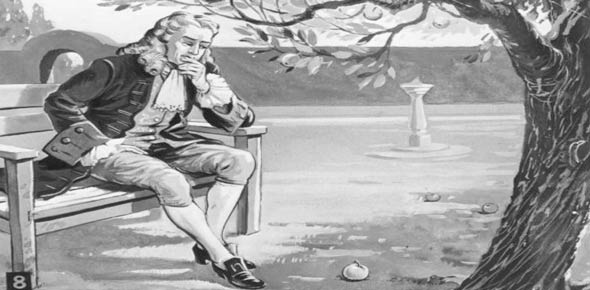# Physics Quiz: Newton's Laws Of Motion

8 Questions | Total Attempts: 13614SettingsWhat do you know about Newton's laws of motion? Isaac Newton is known as the father of physic and is known for the three laws of motion. The law generally describes what happens to an object when force is exerted on it. How well did you know the three laws and how they are applied? Take up the quiz below and get to test your understanding. All the best!

• 1.
1.Every action or force upon an object must have an equal and _________________ reaction.
• A.

Measurable

• B.

Complete

• C.

Opposite

• D.

Correct

• E.

Directionless

• 2.
1.In a vacuum, you throw a baseball. It travels at the __________________ forever due to __________________.
• A.

Initial velocity, inertia

• B.

Gravitational constant velocity, inertia

• C.

Initial velocity, gravity

• D.

Initial acceleration, inertia

• E.

Initial acceleration, gravity

• 3.
When a box rests on the floor, it is acted upon by the gravitational and the _________________ force.
• A.

Centrifugal

• B.

Acceleration

• C.

Normal

• D.

Inertia

• E.

Friction

• 4.
1.Objects in motion will __________________ until acted upon by another force.
• A.

Remain in motion

• B.

Lose potential energy

• C.

Lose kinetic energy

• D.

Lose both potential and kinetic energy

• E.

None of the above

• 5.
According to Newton, force is equal to mass multiplied by __________________.
• A.

Velocity

• B.

Acceleration

• C.

Speed

• D.

Momentum

• E.

Vector plane

• 6.
1.On Earth, you weigh 80 kg. On Planet X, you weigh 20 kg. This is explained due to:
• A.

Different gravitational constants

• B.

Different air resistance

• C.

Water weight

• D.

Different frictional forces

• E.

None of the above

• 7.
1.Which of the following is not an example of acceleration?
• A.

Turning right onto another street

• B.

Pressing the gas pedal

• C.

Pressing the brake pedal

• D.

Cruising at 60 MPH

• E.

Pulling the car into reverse from rest

• 8.
1.Which of the following helps to explain why it takes longer for a piece of paper to fall down than a rock?
• A.

Gravitational force depends on mass

• B.

Gravitational force depends on initial speed

• C.

Paper is lighter than rock

• D.

Paper has a greater surface area than rock

• E.

Rock is slightly magnetic

Related TopicsBack to top